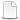HomeTechniques and Tips@RISK Simulation: Numerical ResultsSemivariance, Semideviation, Mean Absolute Deviation

# 6.12. Semivariance, Semideviation, Mean Absolute Deviation

Applies to: @RISK for Excel 5.x–7.x

Can @RISK compute upper and lower semivariance, semideviation, and mean absolute deviation?

Yes, beginning with @RISK 7.5 you can use @RISK statistic functions to compute these quantities automatically. In all statistic functions, datasource can be the name of an input or output, in quotes, or a cell reference.

• Lower semivariance: RiskSemiVariance(datasource) or RiskSemiVariance(datasource, TRUE, simnumber)
• Upper semivariance: RiskSemiVariance(datasource, FALSE) or RiskSemiVariance(datasource, FALSE, simnumber)
(Upper semivariance plus lower semivariance equals variance.)
• Lower semideviation: RiskSemiStdDev(datasource) or RiskSemiStdDev(datasource, TRUE, simnumber)
• Upper semideviation: RiskSemiStdDev(datasource, FALSE) or RiskSemiStdDev(datasource, FALSE, simnumber)
(Lower and upper semideviation are square roots of lower and upper semivariance. The sum of lower and upper semideviations doesn't equal the standard deviation.)
• Mean absolute deviation: RiskMeanAbsDev(datasource) or RiskMeanAbsDev(datasource, simnumber)
(Lower and upper mean absolute deviation are each half of the mean absolute deviation.)

But I have an earlier version of @RISK, and I'm required to use this version. Is there a workaround?

In earlier versions, you can do it yourself with user-defined functions in VBA, or by manipulating the iteration data with RiskData( ) functions. The attached file illustrates both approaches, and shows that they have the same result. (To call on @RISK in user-defined functions in VBA, you need @RISK Industrial or Professional. @RISK Standard Edition does not support automating @RISK.)

Lower and upper semivariance are computed in a similar way to variance: take the sum of squares of differences from the mean, and divide by number of iterations minus 1. (The minus 1 is necessary to create an unbiased estimate of variance, because the simulation is a sample, not the whole population.) However, in computing lower semivariance, use 0 in place of squared deviations above the mean; and in computing upper semivariance, use 0 in place of squared deviations below the mean. Equations might make this clearer:where n is the number of iterations, and IF(condition) has the value 1 if condition is true and 0 if it is false.

Notice that values on the "wrong" side of the mean are not simply omitted; rather, they are replaced by zeroes, so the denominator of the semivariance is the same as the denominator of the variance. Though some authors replace n with the number of values lower (higher) than the mean for lower (upper) semivariance, this article follows Estrada, Rohatgi, and others. Thus the sum of lower and upper semivariance is the variance.

Lower and upper semideviation are found by taking the square roots of lower and upper semivariance. The sum of lower and upper semideviations is of course different from the standard deviation of the full sample.

Lower and upper mean absolute deviation (MAD) are found by taking the sum of the absolute values of deviations from the mean, divided by the number of iterations. However, in computing lower MAD, use 0 in place of deviations above the mean; and in computing upper MAD, use 0 in place of deviations below the mean. Lower and upper mean absolute deviation are numerically equal for any simulated data set, and each is equal to half of the plain mean absolute deviation.

•Semivariance_KB170.xlsm